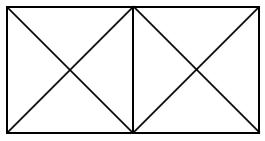Question 1

# How many triangles are there in the following figure?Solution

Each square inside rectangular shape contain 4 small triangle and 4 medium triangle,a total of 8 triangle.

And rectangular shape has 2 large triangle.

So, a total of$$(2×8+2=18)$$ triangles are there in the shape.

C is correct choice.